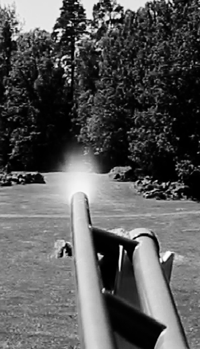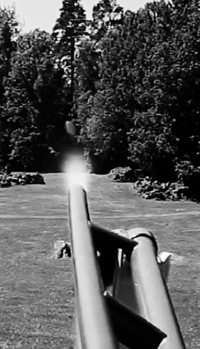﻿ Kartoffelkanon

## The physics behind the pneumatic cannon### Theoretical calculation of the muzzle velocity

Doing calculations on the potato cannon involves a lot of physical concepts, which we will review in the following.

The potato cannon works by compressed air propelling a projectile. The compressed air is situated in a pressure vessel with a volume $V_0$.We denote the initial pressure in the tank by $p_{0}$ and the barometric pressure by $B$.

(The pressure which we read from the pressure gauge is the overpressure $p_{0} - B$. Thus, the pressure in the cylinder is always about 1 bar greater than the manometer read-out of the gun).

As the pressure on one side of the projectile is greater than the pressure on the other side of the projectile, this gives rise to a force which forces the bullet out of the tube. As soon as the projectile is out of the tube, there will be a pressure equalization, so that the forcing stops. At this point, the projectile velocity is $v$.

Pressure is defined by

$$p = \frac{F}{A}$$

whereby

$$F = p \cdot A$$

Here $A$ is the pipe cross sectional area.

We can now create a force diagram for the projectile.The frictional force between the projectile and the tube we denote $F_{\mu}$. The force of the air in the container is $F$ and the power of the atmospheric air in the open end of the pipe is $F_{\text{atm}}$.

While

$$F_{\text{atm}} = A \cdot B$$

is constant, the compressive force

$$F = A \cdot p$$

decreases out through the pipe as the pressure $p$ in the reservoir decreases with the length of the pipe $x$.

Newton's 2nd Law gives us

\begin{equation} m \cdot a = F - F_{\text{atm}} - F_{\mu} \end{equation}

or in differential form

$$m \cdot \frac{\mathrm{d}v}{\mathrm{d}t} = A \cdot p - A \cdot B - F_{\mu}$$

Now

$$\frac{\mathrm{d}v}{\mathrm{d}t} = \frac{\mathrm{d}x}{\mathrm{d}x} \cdot \frac{\mathrm{d}v}{\mathrm{d}t} = \frac{\mathrm{d}x}{\mathrm{d}t} \cdot \frac{\mathrm{d}v}{\mathrm{d}x} = v \cdot \frac{\mathrm{d}v}{\mathrm{d}x}$$

so we can express (1) as

\begin{equation}m \cdot v \cdot \frac{\mathrm{d}v}{\mathrm{d}x} = A \cdot p - A \cdot B - F_{\mu}\end{equation}

This differential equation is solvable if we can express the pressure $p$ as a function of $x$.

When we open the valve, the air in the container expand.Assuming that the air is expanding so rapidly that there is no heat exchange with the environment (the gun and the projectile), we can use the formula for an adiabatic expansion of an ideal gas

$$p_0 \cdot V_0^{\gamma} = p \cdot V^{\gamma} \,\,\,\,\,\,\,\,\, \text{, for luft er } \gamma = 1,4$$

So we have

\begin{equation}p = p_0 \cdot \left(\frac{V_0}{V}\right)^{\gamma}\end{equation}

The figure shows that $V = V_0 + \Delta V$, where $\Delta V$ is the increased volume of air in the container, and when

$$\Delta V = A \cdot x$$

we can express (3) as

$$p = p_0 \cdot \left(\frac{V_0}{V_0 + A \cdot x}\right)^{\gamma}$$

Since we now have expressed $p$ as a function of $x$, we can insert this expression into (2). Then we get

$$m \cdot v \cdot \frac{\mathrm{d}v}{\mathrm{d}x} = A \cdot p_0 \cdot \left(\frac{V_0}{V_0 + A \cdot x}\right)^{\gamma} - A \cdot B - F_{\mu}$$

By separation of the variables, we get after a little rewriting

$$m \cdot \int \!v \, \mathrm{d}v = \int \left( A \cdot p_0 \cdot V_0^{\gamma} \cdot \left( V_0 + A \cdot x \right)^{- \gamma} - A \cdot B - F_{\mu} \right) \, \mathrm{d}x$$

Now we integrate over the length of the pipe

$$m \cdot \int\limits_{0}^{v_{\text{end}}} \!v \, \mathrm{d}v = \int\limits_{0}^{L} \left( A \cdot p_0 \cdot V_0^{\gamma} \cdot \left( V_0 + A \cdot x \right)^{- \gamma} - A \cdot B - F_{\mu} \right) \, \mathrm{d}x$$

that is

\begin{equation}m \cdot \int\limits_{0}^{v_{\text{end}}} \!v \, \mathrm{d}v = A \cdot p_0 \cdot V_0^{\gamma} \cdot \int\limits_{0}^{L} \left( V_0 + A \cdot x \right)^{- \gamma} \, \mathrm{d}x - \int\limits_{0}^{L} A \cdot B \, \mathrm{d}x - \int\limits_{0}^{L} F_{\mu} \, \mathrm{d}x \end{equation}

From mathematics it is well known that

$$\int \!(ax+b)^n \, \mathrm{d}x = \frac{(ax+b)^{n+1}}{(n+1) \cdot a}$$

then by integration of (4) we get

$$\frac{1}{2} \cdot m \cdot v_{\text{end}}^2 = A \cdot p_0 \cdot V_0^{\gamma} \cdot \bigg[ \frac{(V_0+A \cdot x)^{-\gamma +1}}{(- \gamma +1) \cdot A} \bigg]_{0}^{L} -A \cdot B \cdot L - F_{\mu} \cdot L$$

and thereby

$$\frac{1}{2} \cdot m \cdot v_{\text{end}}^2 = A \cdot p_0 \cdot V_0^{\gamma} \cdot \left( \frac{(V_0+A \cdot L)^{1-\gamma}}{(1- \gamma) \cdot A} - \frac{V_0^{1-\gamma}}{(1-\gamma) \cdot A}\right) -A \cdot B \cdot L - F_{\mu} \cdot L$$

or

$$\frac{1}{2} \cdot m \cdot v_{\text{end}}^2 = \frac {\cancel{A} \cdot p_0 \cdot V_0^{\gamma}}{(1- \gamma) \cdot \cancel{A}} \cdot \left( (V_0+A \cdot L)^{1-\gamma} - V_0^{1-\gamma} \right) -A \cdot B \cdot L - F_{\mu} \cdot L$$

We are taking advantage of the fact that $1 - \gamma = - (\gamma - 1)$, and that $V_0^{\gamma} = V_0 \cdot V_0^{\gamma - 1}$. Then vi get

$$\frac{1}{2} \cdot m \cdot v_{\text{end}}^2 = \frac {p_0 \cdot V_0 \cdot V_0^{\gamma-1}}{-(\gamma-1)} \cdot \left( \left( \frac{1}{V_0+A \cdot L}\right)^{\gamma-1} - \left( \frac{1}{V_0}\right)^{\gamma-1} \right) -A \cdot B \cdot L - F_{\mu} \cdot L$$

and again

$$\frac{1}{2} \cdot m \cdot v_{\text{end}}^2 = \frac {p_0 \cdot V_0}{\gamma-1} \cdot \left(1 - \left( \frac{V_0}{V_0+A \cdot L}\right)^{\gamma-1} \right) -A \cdot B \cdot L - F_{\mu} \cdot L$$

Finally we get the desired expression for the muzzle velocity

\begin{equation} \underline{v_{\text{end}} = \sqrt{\frac{2}{m}} \cdot \sqrt{\frac {p_0 \cdot V_0}{\gamma-1} \cdot \left(1 - \left( \frac{V_0}{V_0+A \cdot L}\right)^{\gamma-1} \right) -A \cdot B \cdot L - F_{\mu} \cdot L}}\end{equation}

In practice, the frictional force is very small compared to the pressing force, so we can ignore this force.

### Calculation Example

We can now insert values ​​for the potato cannon in the above expression for the muzzle velocity.

The vessel has an inside diameter of 57 mm and the total pipe length is 3 m So the container volume is

$$V_0 = \frac{\pi}{4} \cdot (0,057\,m)^2 \cdot 3\, m = \underline{0,0077 \,m^3} = 7,7 \, L$$

The inner diameter of the cannon pipe is $d = 45 \, mm$ and its length is $L = 1,8 \, m$. Then the volume of the cannon pipe is

$$A \cdot L = \frac{\pi}{4} \cdot (0,045\,m)^2 \cdot 1,8\, m = \underline{0,0029 \,m^3} = 2,9 \, L$$

As mentioned earlier $\underline{\gamma = 1,4}$ for air.

A typical value for the mass of a potato projectile is $\underline{m = 80 \, \text{g}}$.

Barometric pressure is assumed the default $B = 1 \, \text{atm} = 1,01 \, \text{bar} = \underline{101.000 \, \text{Pa}}$.

The charge pressure (the manometer read-out), we set to 5.0 bar, whereby the initial pressure in the reservoir is

$$p_0 = 5,0 \, \text{bar} + B = 5,0 \, \text{bar} + 1,01 \, \text{bar} = 6,01 \, \text{bar} = \underline{601.000 \, \text{Pa}}$$

If we ignore the frictional force between the projectile and the gun barrel, then with the above values put into (5) we get

\begin{align*} v_{\text{end}} &= \scriptsize \sqrt{\frac{2}{0.080\,\text{kg}}} \cdot \sqrt{\frac {601.000\,\text{Pa} \cdot 0,0077\,\text{m}^3}{0,4} \cdot \left(1 - \left( \frac{0,0077\,\text{m}^3}{0,0077\,\text{m}^3+0,0029\,\text{m}^3}\right)^{0,4} \right) -0,0029\,\text{m}^3 \cdot 101.000\,\text{Pa}} \\ &= \underline{166\,\text{m/s}}\end{align*}

This result for the muzzle velocity is the theoretical maximum value and does not take into account that in practice it is impossible to open the valve immediately. Neither does the theoretical formula take into account that the diameter of the valve hole is less than the diameter of the cannon pipe, which makes the the pressure in the tube being lesser than the pressure in the air container (nozzle effect).

The potato projectile does not fit in perfectly into the cannon tube, which means that some of the air pushes past the potato as the image series below is revealing.